## Delta Function Potentials p.2

Our aim is to solve the time independent Schrödinger's equation to find the probability amplitude (i.e., the wavefunction) as a function of position.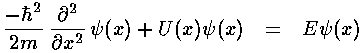where U(x) consists of linear combinations of Dirac delta functions with singular points at various locations. (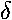(x-a) has its singular point at x=a.)

As usual our first step is to look at units and seek a dimensionless version of Schrödinger's equation. We immediately find an anomaly:is a function which has units (if its argument has units). Look at the fundamental properties of: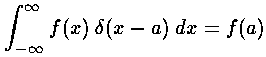f, of course, has the same units on both sides of the equation, so the units ofmust cancel the units of dx...i.e., the units ofare the reciprocal of the units of its argument.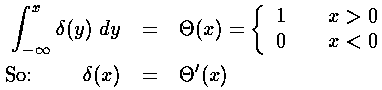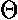is unitless (0 or 1) and so the units of' = d/dx come just from the dx in the denominator. Again the units ofare the reciprocal of the units of its argument.

Thus if we make a potential energy: U(x)=w(x-a),has the units of 1/length (since x has units of length) so w must have units of energy·length.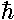2/m=A has the units of energy·length2.

Thus we can make our length scale L=A/w, and our energy scale e=w2/A.

The algebra of this conversion can be a little tricky we note:(x)=(x'·L)= (1/L)(x')

So our dimensionless version of Schrödinger's equation becomes:In the following pages we will drop all primes, so beware that on the following pages x' is called simply x.

Note that our strength of potential w has completely disappeared. It is, of course, still present in our length and energy scale factors. Thus shrinking the dimensionless location of all delta function singularities corresponds to fixed real-space positions with a reduced potential.

Note that we will mostly be considering attractive delta function potentials so our Schrödinger's equation will have -rather than the +shown above.